GeeksforGeeks App
Open AppBrowser
Continue

# Compiler Design – Variants of Syntax Tree

A syntax tree is a tree in which each leaf node represents an operand, while each inside node represents an operator. The Parse Tree is abbreviated as the syntax tree. The syntax tree is usually used when representing a program in a tree structure.

### Rules of Constructing a Syntax Tree

A syntax tree’s nodes can all be performed as data with numerous fields. One element of the node for an operator identifies the operator, while the remaining field contains a pointer to the operand nodes. The operator is also known as the node’s label. The nodes of the syntax tree for expressions with binary operators are created using the following functions. Each function returns a reference to the node that was most recently created.

1. mknode (op, left, right): It creates an operator node with the name op and two fields, containing left and right pointers.

2. mkleaf (id, entry): It creates an identifier node with the label id and the entry field, which is a reference to the identifier’s symbol table entry.

3. mkleaf (num, val): It creates a number node with the name num and a field containing the number’s value, val. Make a syntax tree for the expression a 4 + c, for example. p1, p2,…, p5 are pointers to the symbol table entries for identifiers ‘a’ and ‘c’, respectively, in this sequence.

Example 1: Syntax Tree for the string a – b ∗ c + d is: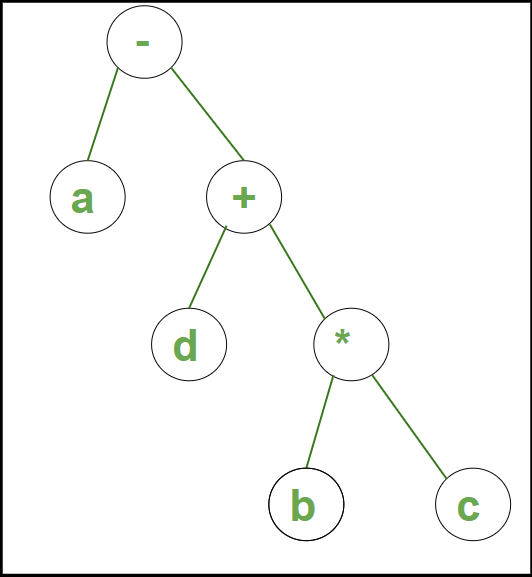Syntax tree for example 1

Example 2: Syntax Tree for the string a * (b + c) – d /2 is: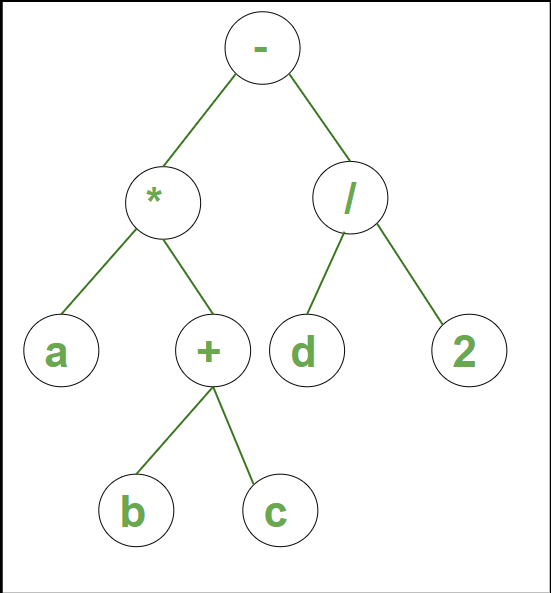Syntax tree for example 2

### Variants of syntax tree:

A syntax tree basically has two variants which are described below:

1. Directed Acyclic Graphs for Expressions (DAG)
2. The Value-Number Method for Constructing DAGs

### Directed Acyclic Graphs for Expressions (DAG)

A DAG, like an expression’s syntax tree, includes leaves that correspond to atomic operands and inside codes that correspond to operators. If N denotes a common subexpression, a node N in a DAG has many parents; in a syntax tree, the tree for the common subexpression would be duplicated as many times as the subexpression appears in the original expression. As a result, a DAG not only encodes expressions more concisely but also provides essential information to the compiler about how to generate efficient code to evaluate the expressions.

The Directed Acyclic Graph (DAG) is a tool that shows the structure of fundamental blocks, allows you to examine the flow of values between them, and also allows you to optimize them. DAG allows for simple transformations of fundamental pieces.

Properties of DAG are:

1. Leaf nodes represent identifiers, names, or constants.
2. Interior nodes represent operators.
3. Interior nodes also represent the results of expressions or the identifiers/name where the values are to be stored or assigned.

Examples:

```T0 = a+b   --- Expression 1
T1 = T0 +c  --- Expression 2```

Expression 1: T0 = a+b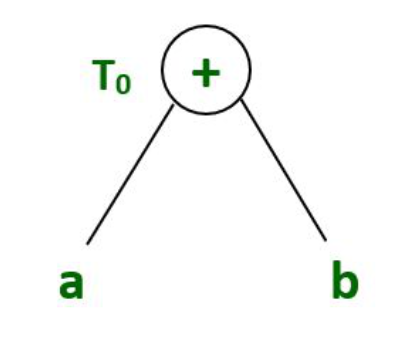Syntax tree for expression 1

Expression 2: T1 = T0 +c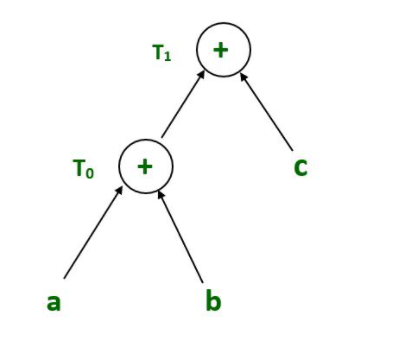Syntax tree for expression 2

#### The Value-Number Method for Constructing DAGs:

An array of records is used to hold the nodes of a syntax tree or DAG. Each row of the array corresponds to a single record, and hence a single node. The first field in each record is an operation code, which indicates the node’s label. In the given figure below,  Interior nodes contain two more fields denoting the left and right children, while leaves have one additional field that stores the lexical value (either a symbol-table pointer or a constant in this instance).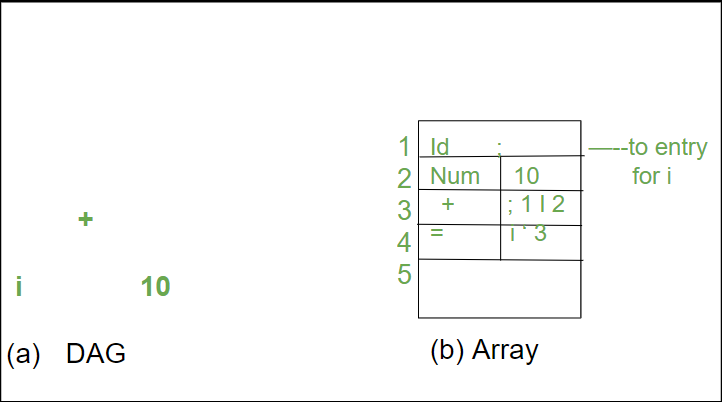Nodes of a DAG for i = i + 10 allocated in an array

The integer index of the record for that node inside the array is used to refer to nodes in this array. This integer has been referred to as the node’s value number or the expression represented by the node in the past. The value of the node labeled -I- is 3, while the values of its left and right children are 1 and 2, respectively. Instead of integer indexes, we may use pointers to records or references to objects in practice, but the reference to a node would still be referred to as its “value number.” Value numbers can assist us in constructing expressions if they are stored in the right data format.

• Algorithm: The value-number method for constructing the nodes of a Directed Acyclic Graph.
• INPUT: Label op, node /, and node r.
• OUTPUT: The value number of a node in the array with signature (op, l,r).
• METHOD: Search the array for node M with label op, left child I, and right child r. If there is such a node, return the value number of M. If not, create in the array a new node N with label op, left child I, and right child r, and return its value number.

While Algorithm produces the intended result, examining the full array every time one node is requested is time-consuming, especially if the array contains expressions from an entire program. A hash table, in which the nodes are divided into “buckets,” each of which generally contains only a few nodes, is a more efficient method. The hash table is one of numerous data structures that may effectively support dictionaries. 1 A dictionary is a data type that allows us to add and remove elements from a set, as well as to detect if a particular element is present in the set. A good dictionary data structure, such as a hash table, executes each of these operations in a constant or near-constant amount of time, regardless of the size of the set.

To build a hash table for the nodes of a DAG, we require a hash function h that computes the bucket index for a signature (op, I, r) in such a manner that the signatures are distributed across buckets and no one bucket gets more than a fair portion of the nodes. The bucket index h(op, I, r) is deterministically computed from the op, I, and r, allowing us to repeat the calculation and always arrive at the same bucket index per node (op, I, r).

The buckets can be implemented as linked lists, as in the given figure. The bucket headers are stored in an array indexed by the hash value, each of which corresponds to the first cell of a list. Each column in a bucket’s linked list contains the value number of one of the nodes that hash to that bucket. That is, node (op,l,r) may be located on the array’s list whose header is at index h(op,l,r).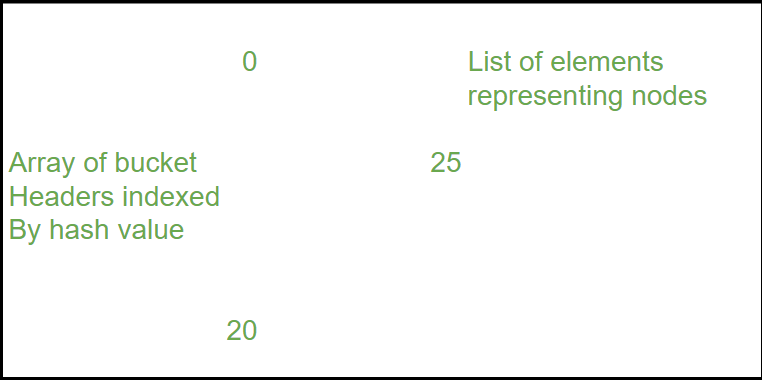Data structure for searching buckets

We calculate the bucket index h(op,l,r) and search the list of cells in this bucket for the specified input node, given the input nodes op, I, and r. There are usually enough buckets that no list has more than a few cells. However, we may need to examine all of the cells in a bucket, and for each value number v discovered in a cell, we must verify that the input node’s signature (op,l,r) matches the node with value number v in the list of cells (as in fig above). If a match is found, we return v. We build a new cell, add it to the list of cells for bucket index h(op, l,r), and return the value number in that new cell if we find no match.

My Personal Notes arrow_drop_up